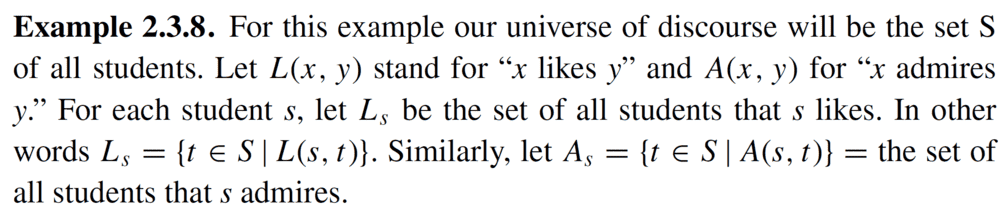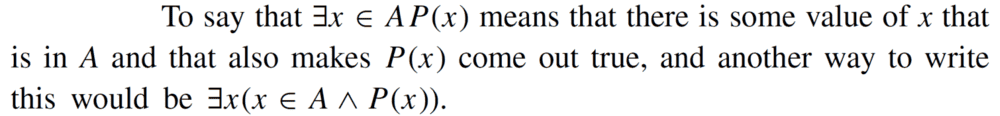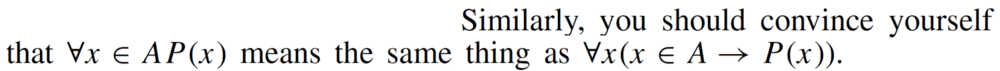# Union of sets and indexed sets question from Vellerman

• CGandC
In summary, the question is asking to describe the set ## \bigcup_{s \in S} L_{s} \, \backslash \, \bigcup_{s \in S} A_{s} ## and the answer is ## \{t\,| t \in \bigcup_{s \in S} L_{s} \, \land \, t \notin \bigcup_{s \in S} A_{s} \} \, ##, which can also be written as ## \forall t ( [ ( \exists s \in S \, L(s,t) ) \land ( t \in S ) ] \land [\neg

#### CGandC

Homework Statement:: x
Relevant Equations:: x

I stumbled upon the following example in the book - " How to prove it, A structured approach " ( 2nd edition) , Vellerman.

Homework Statement::He then asks to describe the set:
## \bigcup_{s \in S} L_{s} \, \backslash \, \bigcup_{s \in S} A_{s} ##

Relevant Equations::
## L_{s} = \{ t \in S | \, L(s,t) \} ##
## A_{s} = \{ t \in S | \, A(s,t) \} ##

and It was also defined beforehand in the book that:
## \bigcup_{s \in S} L_{s} = \{t\,| \exists s \in S \, ( t \in L_{s} ) \} \, = \, \{t \in S | \, \exists s \in S \, L(s,t) \} ##
## \bigcup_{s \in S} A_{s} = \{t\,| \exists s \in S \, ( t \in A_{s} ) \} \, = \, \{t \in S | \, \exists s \in S \, A(s,t) \} ##

## \bigcup_{s \in S} L_{s} \, \backslash \, \bigcup_{s \in S} A_{s} = \{t\,| t \in \bigcup_{s \in S} L_{s} \, \land \, t \notin \bigcup_{s \in S} A_{s} \} \, ##

- - - - - - - - - - - - - - - - - - - - - - - - - - - - - - - - - - - - - -- - - - - - - - - - - - - -- - - - - - - - - - - - - -
Attempt at a solution::
I have arrived to the same answer, however, I have decided to venture further and write the statement logically in its most concise form,
so the following is my answer, I would highly appreciate if you could tell me if I was correct in my reasoning and my answer as written below:

Given:
## L_{s} = \{ t \in S | \, L(s,t) \} ##
## A_{s} = \{ t \in S | \, A(s,t) \} ##

It is also known that:
## \bigcup_{s \in S} L_{s} = \{t\,| \exists s \in S \, ( t \in L_{s} ) \} \, = \, \{t \in S | \, \exists s \in S \, L(s,t) \} ##
## \bigcup_{s \in S} A_{s} = \{t\,| \exists s \in S \, ( t \in A_{s} ) \} \, = \, \{t \in S | \, \exists s \in S \, A(s,t) \} ##

I write the last statements as:
## \bigcup_{s \in S} L_{s} = \{t\,| ( \exists s \in S \, L(s,t) ) \land ( t \in S ) \} \, ##
## \bigcup_{s \in S} A_{s} = \{t\,| ( \exists s \in S \, A(s,t) ) \land ( t \in S ) \} \, ##

Thus
## \bigcup_{s \in S} L_{s} \, \backslash \, \bigcup_{s \in S} A_{s} = \{t\,| t \in \bigcup_{s \in S} L_{s} \, \land \, t \notin \bigcup_{s \in S} A_{s} \} \, ## = ## \forall t ( t \in \bigcup_{s \in S} L_{s} \, \land \, t \notin \bigcup_{s \in S} A_{s} ) ##
## = \forall t ( [ ( \exists s \in S \, L(s,t) ) \land ( t \in S ) ] \land \neg [( \exists s \in S \, A(s,t) ) \land ( t \in S ) ] ) ##
(changing the bound variable from s to b in the second half of the expression to avoid confusion, thus: ) ## = \forall t ( [ ( \exists s \in S \, L(s,t) ) \land ( t \in S ) ] \land \neg [( \exists b \in S \, A(b,t) ) \land ( t \in S ) ] ) ##
( using the identity ## \neg ( P \land Q ) = \neg P \lor \neg Q ## , thus: )
## = \forall t ( [ ( \exists s \in S \, L(s,t) ) \land ( t \in S ) ] \land [\neg( \exists b \in S \, A(b,t) ) \lor ( t \notin S ) ] ) ##
( using the distributivity property, thus: )
## = \forall t ( [ ( \exists s \in S \, L(s,t) ) \land ( t \in S ) \land \neg( \exists b \in S \, A(b,t) ) ] \lor [ ( \exists s \in S \, L(s,t) ) \land ( t \in S ) \land ( t \notin S ) ] ) ##
## = \forall t ( ( \exists s \in S \, L(s,t) ) \land ( t \in S ) \land \neg( \exists b \in S \, A(b,t) ) ) ##

In english, this means: the set of all students who are liked by at least one student, but are not admired by any students.

----------------

Was I correct in writing the final logical form of the answer? ( I can't verify this as I'm self-studying )

Here is how I would try to write it. Note that I am also far from proficient on these topics and also learning by trial and error (basically by trying out things).

=========================

So we want to write:
## \bigcup_{s \in S} L_{s} \, \backslash \, \bigcup_{s \in S} A_{s} ##

And we have:
## L_{s} = \{ t \in S \,| \, L(s,t) \} ##
## A_{s} = \{ t \in S \,| \, A(s,t) \} ##
## \bigcup_{s \in S} L_{s} = \, \{t \in S \, | \, \exists s \in S \, [ L(s,t)] \} ##
## \bigcup_{s \in S} A_{s} = \, \{t \in S \, | \, \exists s \in S \, [A(s,t)] \} ##

## \bigcup_{s \in S} L_{s} \, \backslash \, \bigcup_{s \in S} A_{s} = \{t\,| t \in \bigcup_{s \in S} L_{s} \, \land \, t \notin \bigcup_{s \in S} A_{s} \} \, ##

=========================

So, first of all, since the universe of discourse is given as ##S##, it seems to me that we can simplify some of the expressions/definitions (in this specific case). For example, by writing:
## L_{s} = \{ t \, | \, L(s,t) \} ##
## A_{s} = \{ t \, | \, A(s,t) \} ##
## \bigcup_{s \in S} L_{s} = \, \{t \, | \, \exists s \in S \, [ L(s,t)] \} ##
## \bigcup_{s \in S} A_{s} = \, \{t \, | \, \exists s \in S \, [A(s,t)] \} ##

So we have:
## \bigcup_{s \in S} L_{s} \, \backslash \, \bigcup_{s \in S} A_{s} = \{t\,| t \in \bigcup_{s \in S} L_{s} \, \land \, t \notin \bigcup_{s \in S} A_{s} \} \, ##
##= \{t\,| t \in \bigcup_{s \in S} L_{s} \,\land \, \neg \, t \in \bigcup_{s \in S} A_{s} \} \, ##
##= \{t\,| \, \exists s \in S \, [L(s,t)] \,\land \, \neg \, \exists s \in S \, [A(s,t)] \} \, ##
##= \{t\,| \, \exists s \in S \, [L(s,t)] \,\land \, \neg \, \exists b \in S \, [A(b,t)] \} \, ##
##= \{t\,| \, \exists s \in S \, [L(s,t)] \,\land \, \, \forall b \in S \, [\neg \, A(b,t)] \} \, ##
##= \{t\,| \, \exists s \, [L(s,t)] \,\land \, \, \forall b \, [\neg \, A(b,t)] \} \, ##

Last edited:
One other point which is not directly related to question in OP but is relevant (in slightly different cases) and useful to know.

Suppose ##S## is our domain of discourse (to keep in line with OP). Then when we write:
##\forall x \in S \, (P(x))##
it just simplifies to:
##\forall x \, (P(x))##

Now suppose we have ##B \subset S##. As was discussed in the thread you made, we have:
##\forall x \in B \, (P(x))##
equivalent to both of the following:
##\forall x \in S \, (x\in B \rightarrow P(x))##
##\forall x \, (x\in B \rightarrow P(x))##

========================

Again assume ##S## is our domain of discourse. Now, as in the question, if we have an existence statement like
##\exists x \in S \, (P(x))##
it just simplifies to:
##\exists x \, (P(x))##

However it may be the case that our existence statement is slightly different. For example, with ##B \subset S##, suppose it is like:
##\exists x \in B \, (P(x))##
In that case, it is equivalent to both of the following:
##\exists x \in S \, (x\in B \land P(x))##
##\exists x \, (x\in B \land P(x))##

SSequence said:
##= \{t\,| \, \exists s \, [L(s,t)] \,\land \, \, \forall b \, [\neg \, A(b,t)] \} \, ##
I agree, this is all valid if I know that my bound variables belong to the universe of discourse S. However, If there wasn't a single universe but multiple, then it must be stated explicitly somewhere in the statement which bound variable refers to which universe.

Regarding the later comment:
I understand. Even Vellerman writes the following:and also he writes:So there's importance to being careful when expanding either ## \forall x \in A \, P(x) ## or ## \exists x \in A \, P(x) ## , since:
## \forall x \in A \, P(x) ## means ## \forall x ( x \in A \rightarrow P(x) ) ##
## \exists x \in A \, P(x) ## means ## \exists x ( x \in A \land P(x) ) ##
So it is important what the quantifier preceding the x is, since it can mean a lot of difference even in expanded logical syntax of the statement.

CGandC said:
I agree, this is all valid if I know that my bound variables belong to the universe of discourse S. However, If there wasn't a single universe but multiple, then it must be stated explicitly somewhere in the statement which bound variable refers to which universe.
You are right that this is dependent on context. This happens quite often.

As one example, suppose ##\alpha## is some specific ordinal. Then we may wish to refer to some set of ordinals ##S## which is defined by the property:
##S=\{x\,|\,x+\omega<\alpha\}##
Now if were in a context where we were in a context exclusively involving ordinals only, this notation wouldn't be unreasonable. But if that's not the case then we would usually have to specify that ##x## belongs to the class of ordinals. So the general way to write the set ##S## would be something like:
##S=\{x \in \mathrm{Ord} \,|\,x+\omega<\alpha\}##

So we exclusively mention that ##x## belongs to the class of ordinals.

Last edited: